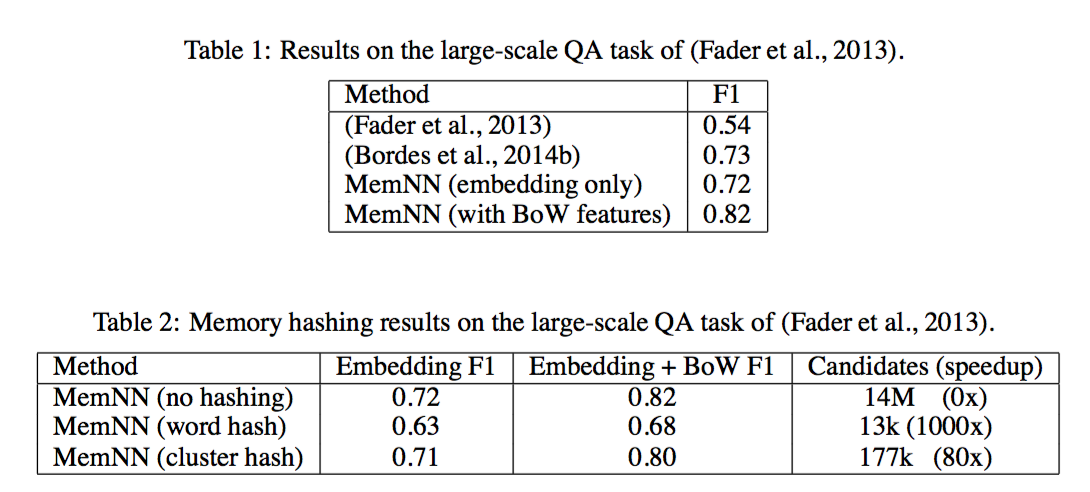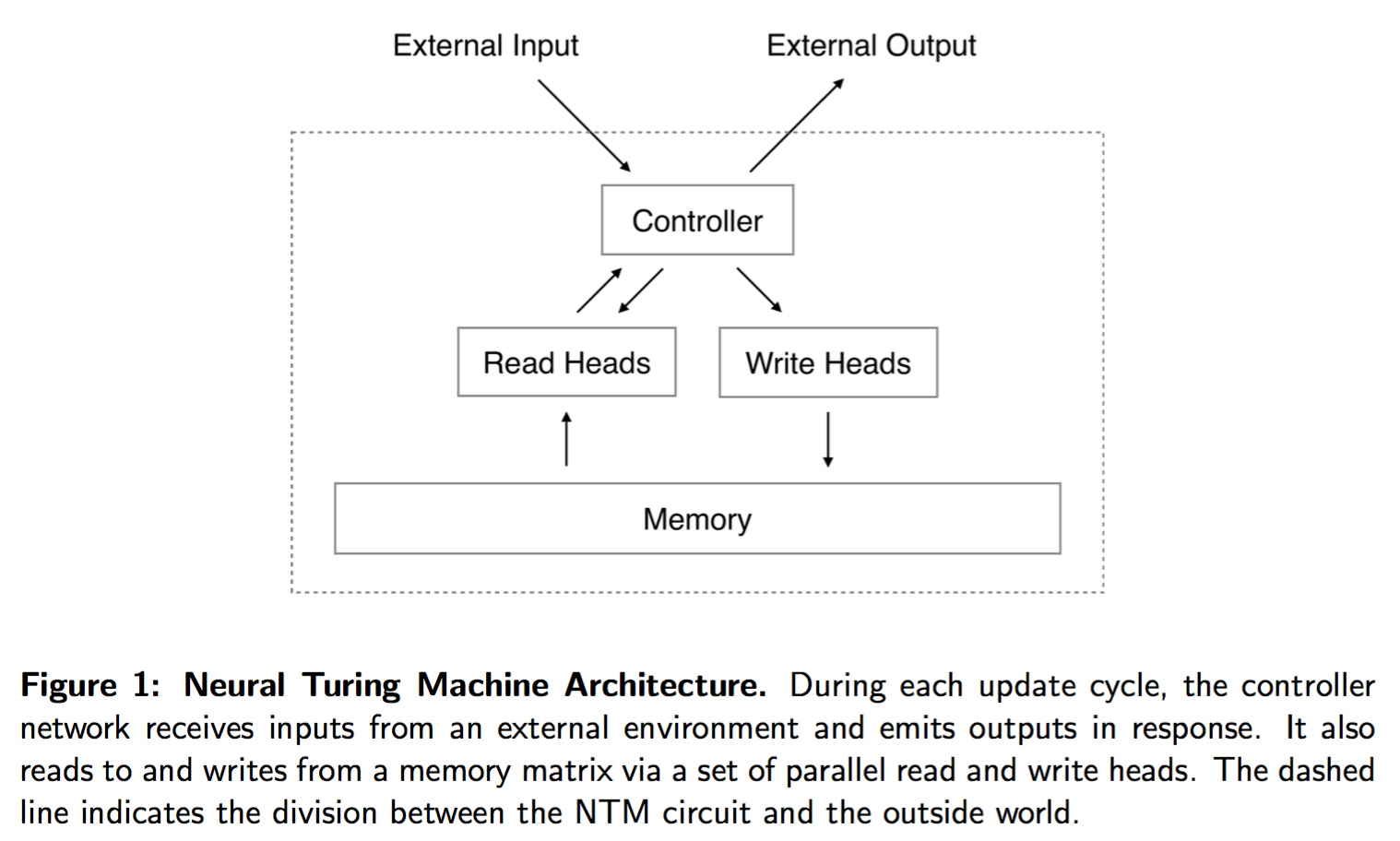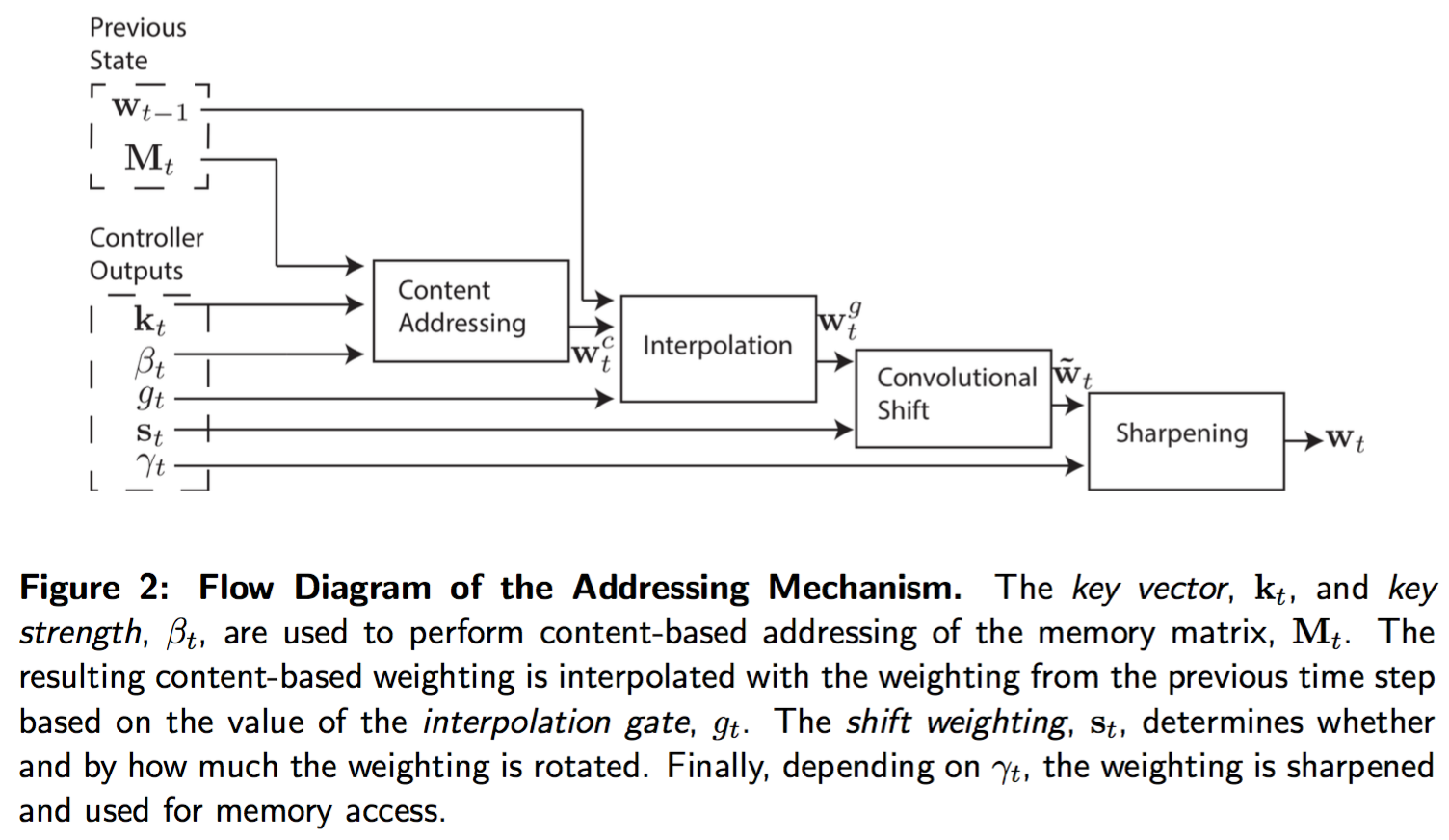Memory Network

## 关于

RNN相当于给神经网络加了记忆单元，做个类比，普通的前馈神经网络（DNN，CNN）就好像是组合逻辑电路；

## Memory Network

•  Memory Network, Jason Weston, Sumit Chopra & Antoine Bordes, Facebook AI Research 2015.
•  End-To-End Memory Networks, Sainbayar Sukhbaatar, Arthur Szlam, Jason Weston, Rob Fergus 2015.

RNN 的缺点在于记忆单元太小，所以记忆能力很弱，比如最简单的问题：输入一串单词，输出刚看到的单词，RNN都难以实现(Zaremba & Sutskever, 2014)

1. I : input feature map, 将输入转换为中间特征表达。
2. G : generalization, 根据新的输入更新存储单元的内容。
3. O : output feature map, 根据当前的输入和存储单元内容，生成输出结果的特征空间表达。
4. R : response, 将输出的特征空间表达转换为目标形式，例如一个文本，或者一个动作。

1. 首先将输入转换为中间表达 $I(x)$
2. 对新的输入x，更新内存 $m_i = G(m_i, I(x), m), \forall i$
3. 对新的输入x 和内存m，计算输出向量 $o = O(I(x), m)$
4. 最后解码输出序列 r = R(o)

## A MemoryNN Implement for Text

### 基本模型

$$o_j = O_j(x, m) = \arg\max_{i=1,...,N} s_O([x, m_{o_1}...,m_{o_{i-1}}], m_i)$$

$$r = \arg\max_{w \in W} s_R([x, m_{o_1},..,m_{o_k}], w)$$

$$s(x, y) = \phi_x(x)^T U^T U \phi_y(y)$$

U 是要学习的参数，$\phi$是对输入的 embedding 函数。

### 训练

1. 选择最匹配的记忆单元带来的风险
2. 选择最佳response带来的风险，如果用RNN就直接用RNN的损失函数就行

### 词序列作为输入

$$seg(c) = W_{swg}^T U_S \Phi_{seg}(c)$$

### EFFICIENT MEMORY VIA HASHING

1. 直接 hash 词，因此对于一个句子，只需比较至少共享一个词的 memory
2. 对词向量做 k-means 聚类，对一个句子，比较的是和至少一个词在同一个类别的memory，这种方法考虑了词的语义。

### MODELING WRITE TIME

$$s(x, y) = \phi_x(x)^T U_{O_t}^T U_{O_t} (\phi_y(y) - \phi_y(y') + \phi_t(x, y, y') )$$

• whether x older than y,
• whether x older than y',
• whether y older than y',

### 精确匹配

embedding 因为将词降维到低维连续向量，所以无法做精确匹配。

$$s(x, y) = \phi_x(x)^T U^T U \phi_y(y) + \lambda \phi_x(x)^T \phi_y(y)$$

### 结果• 可以看出 只用 embedding 特征和之前最好结果差不多，稍低，但是加上 BOW 特征，效果马上就提升了9个点。
• 不同hash策略的影响，word hash 实现了1000倍的加速但是精度减少很明显，cluster hash 在精度只减少1个点的情况下，实现了80倍的加速。• 可以看到时间特征对提升非常显著
• 输出选取的内存个数k对效果提升也非常显著
• MemNN 完胜 RNN，LSTM

## Neural Turing Machine

RNN 是图灵完备的，即可以实现图灵机的所有操作，即可用图灵机完成的事情，RNN有这种潜能可以解决！

NTM之于RNN，就如图灵机之于有限状态机！最大的区别在于前者有接近无限的存储空间！

NTM 通过 attention 机制实现内存的读取和写入操作。

### 基础研究

working memory 在心理学中用于解释短期信息处理能力

1. Baddeley, A., Eysenck, M., and Anderson, M. (2009). Memory. Psychology Press.
2. Miller, G. A. (1956). The magical number seven, plus or minus two: some limits on our capacity for processing information. Psychological review, 63(2):81.

### Recurrent Neural Networks

• state：隐马尔科夫模型具有有限个离散状态；RNN具有无限个分布式状态
• 状态转移概率：隐马尔科夫模型通过转移概率矩阵建模，依赖于当前状态和当前的输入；RNN通过隐层建模，可以是简单的RNN隐层单元，LSTM单元，GRU，甚至更复杂的多层结构，依赖于当前状态和当前的输入。
• 输出：只依赖于当前的状态，通常用生成模型建模这个条件概率；RNN则用（单层或多层）神经网络建模这个条件概率。
• 模型训练：隐马尔科夫模型根据输出的结果，用维特比算法解码出状态，转移概率和条件概率通过EM算法优化得到（参考语音识别）；RNN则是端到端用梯度下降联合优化所有参数得到。
• LSTM 解决RNN梯度消失和爆炸的问题是通过嵌入一个理想积分器？

• RNN 的一些应用场景：

• 语音识别
• Graves, A., Mohamed, A., and Hinton, G. (2013). Speech recognition with deep recurrent neural networks. In Acoustics, Speech and Signal Processing (ICASSP), 2013 IEEE International Conference on, pages 6645–6649. IEEE.
• Graves, A. and Jaitly, N. (2014). Towards end-to-end speech recognition with recurrent neural networks. In Proceedings of the 31st International Conference on Machine Learn- ing (ICML-14), pages 1764–1772.

### 神经图灵机结构• $\mathbf{M}_t$ $N \times M$ 尺寸的内存矩阵，M是每个内存向量的尺寸，N是内存向量的个数
• $\mathbf{w}_t$ 是读头给出的每个内存的权重向量，他应该满足概率约束条件，非负，和为1.
• 读头读内存后返回的结果为

$$\mathbf{r}_t = \sum_i w_t(i) \mathbf{M}_t(i)$$

$\mathbf{M}_t(i)$ 是行向量，也就是一个内存单元。

• 擦除向量 $\mathbf{e}_t$ M 个元素全为0-1之间，设写入权重为$w_t(i)$内存更新方程为

$$\tilde{\mathbf{M}}_t(i) = \mathbf{M}_{t-1}(i)[\mathbf{1} - w_t(i)\mathbf{e}_t]$$

• add 向量 $\mathbf{a}_t$，用add 向量更新擦出后的内存

$$\mathbf{M}_t(i) = \tilde{\mathbf{M}}_t(i) + w_t(i) \mathbf{a}_t$$

### 寻址机制• 两种基本机制：

1. 基于内容的寻址：找和控制器发出的值最相似的位置，Hopfield networks 1982：简单、可以获取内存的精确值；不适合算术问题，例如计算 $x+y$，寻址跟内容无关
2. 基于位置的寻址，可以看做基于内容的寻址的特例，因为位置也可以看做内容的一部分
• 基于内容的寻址原理：每一个 head（读或者写）先生成一个长度为 $M$ 的 key vector $\mathbf{k}_t$，通过这个向量和内存中的所有向量进行比较，计算相似度 $K[·, ·]$，相似度在所有的内存上归一化，$\beta_t$ 是缩放因子。论文中相似度度量采用向量的余弦相似度

$$w_t^c(i) = \frac{\exp\left(\beta_t K[\mathbf{k}_t, \mathbf{M}_t(i)]\right)}{\sum_j \exp\left(\beta_t K[\mathbf{k}_t, \mathbf{M}_t(j)]\right)}$$

• 基于位置的寻址：每一个 head 生成一个标量 interpolation gate $g_t \in (0, 1)$，利用这个门去控制当前权重向量$\mathbf{w}t^c$ 和历史权重$\mathbf{w}$混合生成门限权重

$$\mathbf{w}_{t}^g = g_t \mathbf{w}_t^c + (1 - g_t) \mathbf{w}_{t-1}$$

$$\tilde{w}_t(i) = \sum_{j=0}^{N-1} w_t^g(j) s(i - j)$$

$$w_t(i) = \frac{\tilde{w}_t(i)^{\gamma_t}}{\sum_j \tilde{w}_t(j)^{\gamma_t}}$$

## 未解决的问题

• 擦除向量$e_t$和add向量$a_t$怎么确定？
• 输入数据如何影响读写头的权重？输入数据和 key vector $k_t$ 有什么关系？